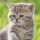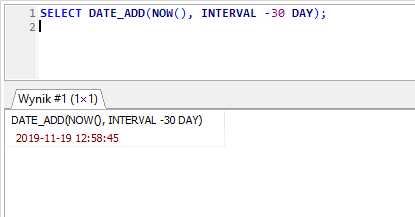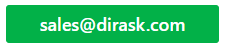Home
IT Knowledge
IT Career
Inspiration
Languages
EN

# MySql - subtract days from current date

16 points
Created by:Kate_C
19800

In MySql, there is available `DATE_ADD()` function that helps to subtract days from some date. In this example we presented way how to subtract some days from current date.

## 1. `DATE_ADD()` with `NOW()` functions example

This example subtracts 30 days from current time.

``SELECT DATE_ADD(NOW(), INTERVAL -30 DAY);``

Result:Subtract 30 days from current date operation in MySql with HeidiSQL.